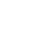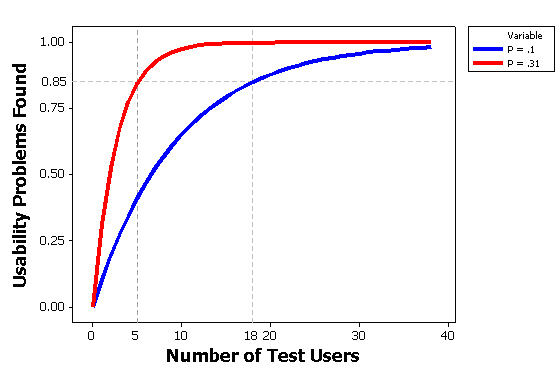# Why you only need to test with five users (explained)

Jeff Sauro, PhD
One question I get a lot is, Do you really only need to test with 5 users? There are a lot of strong opinions about the magic number 5 in usability testing and much has been written about it (e.g. see Lewis 2006PDF). As you can imagine there isn’t a fixed number of users that will always be the right number (us quantitative folks love to say that) but testing with five users may be all you need for discovering problems in an interface, given some conditions.

The five user number comes from the number of users you would need to detect approximately 85% of the problems in an interface, given that the probability a user would encounter a problem is about 31%. Most people either leave off the last part or are not sure what it means. This does not apply to all testing situations such as comparing two products or when trying to get a precise measure of task times or completion rates but to discovering problems with an interface. Where does 31% come from? It was found as an average problem frequency from several studies (more on this below).

5 users only applies to discovering problems, not comparing interfaces or estimating a task time or completion rate.

For example, the calendar on Hertz.com has a problem with the dates. Let’s imagine that this will adversely affect 31% of reservations—which is quite a lot. So the question becomes, if a problem occurs this frequently (affects this many users) how many users do you need to observe to have an 85% chance of seeing it during a usability test? You might be tempted to think you only need 3 to see it once, but chance fluctuations don’t quite work that way at small sample sizes. You actually would need 5, and this comes from the binomial probability.
The formulas actually work quite well, but math tends to bring back bad memories for many people so I’ve provided some simulations below to show you how it works.

### Tossing a Coin (50% Probability)

We all know that there is a 50% chance of getting a tails and 50% chance of getting heads when flipping a coin. If you wanted to know how many times you should plan on flipping a coin to see tails at least once, using the binomial formula the answer is 3. You can see this for yourself; click the flip 1 coin until you see tails. 85% of the time you’ll need to click it no more than three times. You can repeat this exercise and see the number of sample sizes which take more than 3. Over time, a bit more than 85% of all your samples will be 3 or less.

Q: How many times do you need to toss a coin to be 85% sure you’ll see tails at least once?
A: 3 or fewer

On every button click there is a 50% chance you’ll see tails.Sample Sizes :

### Rolling a Die (16.7% Probability)

There is a 1/6 chance of getting any number from a 6-sided die. So on any toss there is a 16.667% chance of getting a 1. The binomial formula predicts that you’d need to toss a die on average 10 times to be 85% sure you’ll see a 1.

Q: How many times do you need to toss a die to be 85% sure you’ll see a one at least once?
A: 10 or fewer

On every button click there is a 16.667% chance you’ll see a one.Sample Sizes :

## UI Problems

Now I have three UI problems which occur 31%, 10% and 1% of the time. Every time you click “Test 1 User” it’s like testing a user (but without the expenses!).

### Detecting Problems that affect 31% of users

Q:How many users do you have to test to be 85% sure you’ll see a problem that affects 31% of users at least once?
A: 5 or fewer

On every button click there is a 31% chance you’ll see a UI Problem.Sample Sizes :

### Detecting Problems that affect 10% of users

Q: How many users do you have to test to be 85% sure you’ll see a problem that affects 10% of users at least once?
A: 18 or fewer

On every button click there is a 10% chance you’ll see a UI Problem.Sample Sizes :

### Detecting Problems that affect 1% of users

Q: How many users do you have to test to be 85% sure you’ll see a problem that affects 1% of users at least once?
A: 189 or fewer

On every button click there is a 1% chance you’ll see a UI Problem.Sample Sizes :

### How many users do I need, then?

So if you plan on testing with five users, know that you’re not likely to see most problems, you just likely to see most problems that affect 31%-100% for this population and set of tasks.

Of course not all problems affect 31% of users. In fact, in released software or websites, the likelihood of encountering a problem might be closer to 10% or 5%. When problems are much less likely to be “detected” by users interacting with the software, you need more users to test to have a decent chance of observing them in a usability test. For example, given a problem only affecting 10% of users, you need to plan on testing 18 to have an 85% chance of seeing it once. I’ve graphed the differences in the figure below. The blue line shows problems affecting 10% of users and the red affects 31% of users.Figure 1: Difference in sample sizes needed to have an 85% chance of detecting a problem that affects 10% of users vs. 31% of users. You would need to plan on testing 18 users to have an 85% chance of detecting problems that affect 10% of users.

### Why the controversy?

There’s no concern about the binomial formula (or Poisson equivalent), the controversy is around how frequently UI problems really occur. In reality they aren’t a fixed percent like 31% or 10%, instead these represent an average of problem frequency.

Problems don’t uniformly affect users. 31% is an average frequency from many studies, for already released applications the frequency is probably less than 10%.

Problems in fact do not uniformly affect users, or affect users in an easily predictable way. While it is difficult to know how frequently problems occur, as a general rule, for early designs it will be higher (31% or more) and for applications that are in use with many users it will likely be below 10%. Of course you don’t know what the probability a user will encounter a problem. In fact, you often don’t even know if there is a problem—if you did you’d fix it!

As a strategy, pick some percentage of problem occurrence, say 20%, and likelihood of discovery, say 85%, which would mean you’d need to plan on testing 9 users. After testing 9 users, you’d know you’ve seen most of the problems that affect 20% or more of the users. If you need to be surer of the findings, then increase the likelihood of discovery, for example, to 95%. Doing so would increase your required sample size to 13.

The best strategy is to bring in some set of users, find the problems they have, fix those problems, then bring in another set of users as part of an iterative design and test strategy. In the end, although you’re never testing more than 5 users at a time, in total you might test 15 or 20 users. In fact, this is what Nielsen recommends in his article, not just testing 5 users in total.

So if you plan on testing with five users, know that you’re not likely to see most problems, you are just likely to see most problems that affect 31%-100% of users for this population and set of tasks. You will also pick up some of the problems that affect less than 31% of users– just not 85% of them. For example, a sample size of 5 should pick up about 50% of the problems with likelihoods of occurrence of 15%, 75% of the problems with likelihoods of 25%, and so on. Change the tasks or type of users and you’ll need a new sample of users.

0
0